• 机器学习(六)采样方法 6.1 蒙特卡洛数值积分 计算f(x)f(x)f(x)的积分时，∫baf(x)dx∫abf(x)dx\int_a^bf(x)dx复杂不好求，可采用蒙特卡洛积分来近似 思想：   ∫baf(x)dx=∫baf(x)q(x)q(x)dx∫abf(x)dx=∫abf...
机器学习(六)采样方法

6.1 蒙特卡洛数值积分
计算f(x)$f\left(x\right)$$f(x)$的积分时，∫baf(x)dx${\int }_{a}^{b}f\left(x\right)dx$$\int_a^bf(x)dx$复杂不好求，可采用蒙特卡洛积分来近似
思想：
∫baf(x)dx=∫baf(x)q(x)q(x)dx${\int }_{a}^{b}f\left(x\right)dx={\int }_{a}^{b}\frac{f\left(x\right)}{q\left(x\right)}q\left(x\right)dx$$\int_a^bf(x)dx=\int_a^b\frac{f(x)}{q(x)}q(x)dx$，把q(x)$q\left(x\right)$$q(x)$作为概率分布，f(x)q(x)$\frac{f\left(x\right)}{q\left(x\right)}$$\frac{f(x)}{q(x)}$作为函数
在q(x)$q\left(x\right)$$q(x)$下抽取n个样本，当n足够大时，可以均值1n∑if(xi)q(xi)$\frac{1}{n}\sum _{i}\frac{f\left({x}_{i}\right)}{q\left({x}_{i}\right)}$$\frac{1}{n}\sum_i\frac{f(x_i)}{q(x_i)}$来近似积分

证明：
E(g(X))=∑xg(x)f(x)$E\left(g\left(X\right)\right)=\sum _{x}g\left(x\right)f\left(x\right)$$E(g(X))=\sum_xg(x)f(x)$，已知X的概率分布为f(x)$f\left(x\right)$$f(x)$，g(x)$g\left(x\right)$$g(x)$的概率分布未知，计算g(x)$g\left(x\right)$$g(x)$的期望
令:g∗(x)=g(x)fX(x)${g}^{\ast }\left(x\right)=\frac{g\left(x\right)}{{f}_{X}\left(x\right)}$$g^*(x)=\frac{g(x)}{f_X(x)}$，则有
E(g∗(X))=∫bag∗(x)fX(x)dx=∫bag(x)dx=I(6.1.1)$\begin{array}{}\text{(6.1.1)}& E\left({g}^{\ast }\left(X\right)\right)={\int }_{a}^{b}{g}^{\ast }\left(x\right){f}_{X}\left(x\right)dx={\int }_{a}^{b}g\left(x\right)dx=I\end{array}$E(g^*(X))=\int_a^bg^*(x)f_X(x)dx=\int_a^bg(x)dx=I\tag{6.1.1}
由强大数定律P(limn→∞1N∑Ni=1g∗(Xi)=I)=1$P\left(\underset{n\to \mathrm{\infty }}{lim}\frac{1}{N}\sum _{i=1}^{N}{g}^{\ast }\left({X}_{i}\right)=I\right)=1$$P(\lim_{n\rightarrow \infty}\frac{1}{N}\sum_{i=1}^Ng^*(X_i)=I)=1$，则:
选I′=1N∑Ni=1g∗(Xi)$I\mathrm{\prime }=\frac{1}{N}\sum _{i=1}^{N}{g}^{\ast }\left({X}_{i}\right)$$I\prime=\frac{1}{N}\sum_{i=1}^Ng^*(X_i)$，I′$I\mathrm{\prime }$$I\prime$依概率1收敛到I

蒙特卡洛方法
随机变量X服从概率分布P(x)$P\left(x\right)$$P(x)$，计算f(x)$f\left(x\right)$$f(x)$的期望，只需要不停从p(x)$p\left(x\right)$$p(x)$抽样xi${x}_{i}$$x_i$，对f(xi)$f\left({x}_{i}\right)$$f(x_i)$取平均即可近似f(x)$f\left(x\right)$$f(x)$的期望

均匀分布与Box-Muller变换
在计算机中生成[0,1]之间的伪随机数序列，可以看成均匀分布
伪随机数序列生成方法xn+1=(axn+c) mod m$x_{n+1}=(ax_n+c) \text{ } mod\text{ } m$
Box-Muller 变换 如果随机变量U1,U2${U}_{1},{U}_{2}$$U_1,U_2$独立且服从[0,1]均匀分布则 :
Z0=−2lnU1−−−−−−√cos(2πU2)${Z}_{0}=\sqrt{-2ln{U}_{1}}cos\left(2\pi {U}_{2}\right)$$Z_0=\sqrt{-2lnU_1}cos⁡(2πU_2)$
Z1=−2lnU1−−−−−−√sin(2πU2)${Z}_{1}=\sqrt{-2ln{U}_{1}}sin\left(2\pi {U}_{2}\right)$$Z_1=\sqrt{-2lnU_1}sin⁡(2πU_2)$
Z0，Z1${Z}_{0}，{Z}_{1}$$Z_0，Z_1$独立且服从标准正太分布

6.2 拒绝采样
假设要以分布p(x)$p\left(x\right)$$p(x)$采样，而直接从p(x)$p\left(x\right)$$p(x)$采样较为困难
提议分布 q(x)$q\left(x\right)$$q(x)$，常数k，使得对于任意x，kq(x)≥p(x)$kq\left(x\right)\ge p\left(x\right)$$kq(x)\geq p(x)$，提议分布易于采样在形状接近p(x)$p\left(x\right)$$p(x)$

采样过程如下：
从提议分布q(x)$q\left(x\right)$$q(x)$生成一个数x0${x}_{0}$$x_0$
从均匀分布[0,kq(x0)]$\left[0,kq\left({x}_{0}\right)\right]$$[0,kq(x_0)]$生成一个数u0${u}_{0}$$u_0$
若u0≤p(x0)${u}_{0}\le p\left({x}_{0}\right)$$u_0\leq p(x_0)$，则认为x0${x}_{0}$$x_0$是一个有效样本

缺点：
合适的 q 分布比较难以找到
很难确定一个合理的 k 值。
这两个问题会导致拒绝率很高，无用计算增加。

6.3 重要性采样
p(z)$p\left(z\right)$$p(z)$分布难以直接采样，可以q(z)$q\left(z\right)$$q(z)$分布进行采样
E(f(Zz∼p(z)))==≈∫f(z)p(z)dz∫f(z)p(z)q(z)q(z)dz1L∑i=1Lp(zl)q(zl)f(zl)(6.3.1)$\begin{array}{rl}E\left(f\left({Z}_{z\sim p\left(z\right)}\right)\right)=& \int f\left(z\right)p\left(z\right)dz\\ =& \int f\left(z\right)\frac{p\left(z\right)}{q\left(z\right)}q\left(z\right)dz\\ \text{(6.3.1)}& \approx & \frac{1}{L}\sum _{i=1}^{L}\frac{p\left({z}^{l}\right)}{q\left({z}^{l}\right)}f\left({z}^{l}\right)\end{array}$\begin{align}
E(f(Z_{z\sim p(z)}))=&\int f(z)p(z)dz\notag\\
=&\int f(z)\frac{p(z)}{q(z)}q(z)dz\notag\\
\approx &\frac{1}{L}\sum_{i=1}^L\frac{p(z^l)}{q(z^l)}f(z^l)\tag{6.3.1}
\end{align}
其中rl${r}_{l}$$r_l$被称为importance weight，
rl=p(zl)q(zl)(6.3.2)$\begin{array}{}\text{(6.3.2)}& {r}_{l}=\frac{p\left({z}^{l}\right)}{q\left({z}^{l}\right)}\end{array}$r_l=\frac{p(z^l)}{q(z^l)}\tag{6.3.2}

给定N个单词的语料库，如何以相应概率采样每个单词？
采用接受拒绝采样
遍历一遍语料，在内存里保留词表 word0 N1 word2 N2 … wordk Nk
取提议分布为均匀分布[0,K]q$q$$q$，k取 maxiNiN∗1N$\frac{\underset{i}{max}{N}_{i}}{N}\ast \frac{1}{N}$$\frac{\max_i{N_i}}{N}*\frac{1}{N}$，以均匀分布q采样x，以均匀分布[0,kq(x)]采样u，若u<Nfloor(x)N$u<\frac{{N}_{floor\left(x\right)}}{N}$$u < \frac{N_{floor(x)}}{N}$，则采样wordfloor(x)$wor{d}_{floor\left(x\right)}$$word_{floor(x)}$
展开全文• 转自：http://www.xuebuyuan.com/1409669.htmlcrossvalind交叉验证Generate cross-validation indices 生成交叉验证索引Syntax语法Indices = crossvalind('Kfold', N, K) K折交叉[Train, Test] = crossvalind('...
转自：http://www.xuebuyuan.com/1409669.html         https://blog.csdn.net/bbbeoy/article/details/72967794针对经验风险最小化算法的过拟合的问题，给出交叉验证的方法，这个方法在做分类问题时很常用：一：简单的交叉验证的步骤如下：1、 从全部的训练数据 S中随机选择 中随机选择 s的样例作为训练集 train，剩余的 作为测试集 作为测试集 test。2、 通过对测试集训练 ，得到假设函数或者模型 。3、 在测试集对每一个样本根据假设函数或者模型，得到训练集的类标，求出分类正确率。4，选择具有最大分类率的模型或者假设。这种方法称为 hold -out cross validation 或者称为简单交叉验证。由于测试集和训练集是分开的，就避免了过拟合的现象二：k折交叉验证 k-fold cross validation1、 将全部训练集 S分成 k个不相交的子集，假设 S中的训练样例个数为 m，那么每一个子 集有 m/k 个训练样例，，相应的子集称作 {s1,s2,…,sk}。2、每次从分好的子集中里面，拿出一个作为测试集，其它k-1个作为训练集3、根据训练训练出模型或者假设函数。4、 把这个模型放到测试集上，得到分类率。5、计算k次求得的分类率的平均值，作为该模型或者假设函数的真实分类率。这个方法充分利用了所有样本。但计算比较繁琐，需要训练k次，测试k次。三：留一法  leave-one-out cross validation留一法就是每次只留下一个样本做测试集，其它样本做训练集，如果有k个样本，则需要训练k次，测试k次。留一发计算最繁琐，但样本利用率最高。适合于小样本的情况。crossvalind交叉验证Generate cross-validation indices  生成交叉验证索引Syntax语法Indices = crossvalind('Kfold', N, K) K折交叉[Train, Test] = crossvalind('HoldOut', N, P)[Train, Test] = crossvalind('LeaveMOut', N, M)留M法交叉验证，默认M为1，留一法交叉验证[Train, Test] = crossvalind('Resubstitution', N, [P,Q])[...] = crossvalind(Method, Group, ...)[...] = crossvalind(Method, Group, ..., 'Classes', C)[...] = crossvalind(Method, Group, ..., 'Min', MinValue)部分转载自https://blog.csdn.net/NNNNNNNNNNNNY/article/details/45789323交叉验证是一种随机循环验证方法，它可以将数据样本随机分割成几个子集。交叉验证主要用于评估统计分析或机器学习算法的泛化能力等。 对于第一种在评估机器学习算法的泛化能力时，我们可以选择随机分割后的一部分数据作为训练样本，另一部分作为测试样本。具体实现流程如下：Data = rand(9,3);%创建维度为9×3的随机矩阵样本
indices = crossvalind('Kfold', 9, 3);%将数据样本随机分割为3部分
for i = 1:3 %循环3次，分别取出第i部分作为测试样本，其余两部分作为训练样本
test = (indices == i);
train = ~test;
trainData = Data(train, :);
testData = Data(test, :);
end相应输出结果： 生成的随机矩阵Data: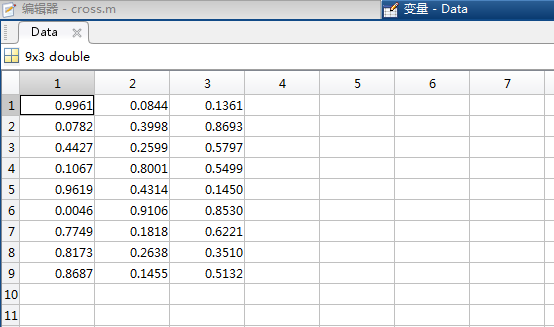indices数据，即分成的三类，数字相同表示对应的行数为同一类：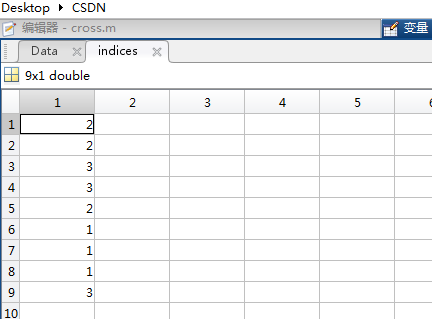当i=3时的test数据：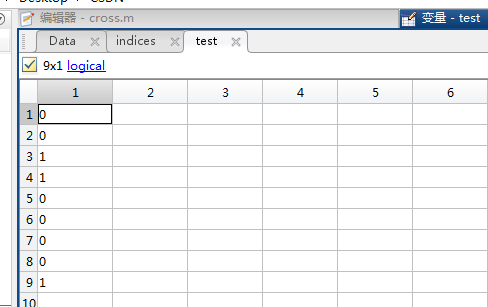对应的train数据（即对test取反）：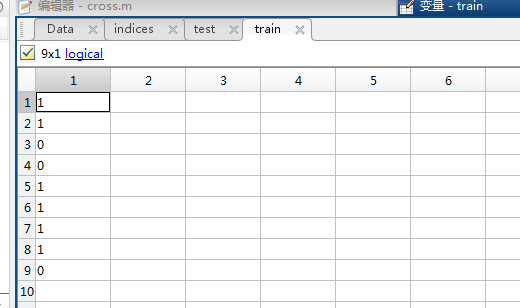testData（即test数据中‘1’所对应的行的数据）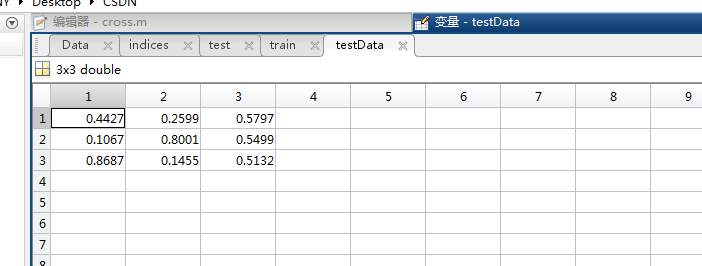trainData：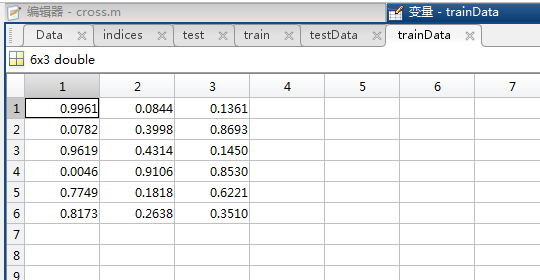k-折交叉验证(k-fold crossValidation)：在机器学习中，将数据集A分为训练集（training set）B和测试集（test set）C，在样本量不充足的情况下，为了充分利用数据集对算法效果进行测试，将数据集A随机分为k个包，每次将其中一个包作为测试集，剩下k-1个包作为训练集进行训练。在matlab中，可以利用：indices=crossvalind('Kfold',x,k);来实现随机分包的操作，其中x为一个N维列向量（N为数据集A的元素个数，与x具体内容无关，只需要能够表示数据集的规模），k为要分成的包的总个数，输出的结果indices是一个N维列向量，每个元素对应的值为该单元所属的包的编号（即该列向量中元素是1~k的整随机数），利用这个向量即可通过循环控制来对数据集进行划分。例：  [M,N]=size(data);//数据集为一个M*N的矩阵，其中每一行代表一个样本
indices=crossvalind('Kfold',data(1:M,N),10);//进行随机分包
for k=1:10//交叉验证k=10，10个包轮流作为测试集
test = (indices == k); //获得test集元素在数据集中对应的单元编号
train = ~test;//train集元素的编号为非test元素的编号
train_data=data(train,:);//从数据集中划分出train样本的数据
train_target=target(:,train);//获得样本集的测试目标，在本例中是train样本的实际分类情况
test_data=data(test,:);//test样本集
test_target=target(:,test);//test的实际分类情况
...........
endcrossvalind函数①indices =crossvalind('Kfold', N, K)： 该命令返回一个对于N个观察样本的K个fold（意为折，有“层”之类的含义，感觉还是英文意思更形象）的标记（indices）。该标记中含有相同（或者近似相同）比例的1—K的值，将样本分为K个相斥的子集。在K-fold交叉检验中，K-1个fold用来训练，剩下的一个用来测试。此过程循环K次，每次选取不同的fold作为测试集。K的缺省值为5。 使用程序： [m n]=size(data); %data为样本集合。每一行为一个观察样本
indices = crossvalind('Kfold',m,10); %产生10个fold，即indices里有等比例的1-10
for i=1:10
test=(indices==i); %逻辑判断，每次循环选取一个fold作为测试集 train=~test; %取test的补集作为训练集，即剩下的9个fold
data_train=data(trian,:); %以上得到的数都为逻辑值，用与样本集的选取 label_train=label(train,:); %label为样本类别标签，同样选取相应的训练集 data_test=data(test,:); %同理选取测试集的样本和标签 label_test=label(test,:); end ②[Train, Test] = crossvalind('HoldOut',N, P)： 该命令返回一个逻辑值的标记向量，从N个观察样本中随机选取（或近似于）P*N个样本作为测试集。故P应为0-1，缺省值为0.5。 使用程序： groups=ismenber(label,1); %label为样本类别标签，生成一个逻辑矩阵groups,1用来逻辑判断筛选
[train, test] = crossvalind('holdOut',groups); %将groups分类，默认比例1:1，即P=0.5 ③[Train, Test] = crossvalind('LeaveMOut',N, M)： 该命令返回一个逻辑值的标记向量，从N个观察样本中随机选取M个样本作为测试集。M的缺省值为1。值得注意的是，LeaveMOut在循环中使用不能保证产生的是互补集合，即每次循环的随机选取是独立的。如果要用互补的话还是使用Kfold命令。 使用程序： [m,n]=size(data);
[train,test]=crossvalind('LeaveMOut',m,10)
svmStruct = svmtrain(data(train,:),groups(train));  classes = svmclassify(svmStruct,data(test,:));  cp=classperf(groups); cr=cp.CorrectRate ④[Train, Test] = crossvalind('Resubstitution',N, [P,Q])：本函数为②的一个特殊情况。当我不想把 P*N 剩下的部分全部作为训练集的时候使用该函数，用 Q 指定一个比例， 选取 Q*N 作为训练集。两个集合的选取以最小化交集为原则。 
展开全文• 机器学习样本比例不平衡时，通常会对数据进行采样的方法，对数据采样可以有针对性地改变数据中样本的比例，采样一般有两种方式：over-sampling 和 under-sampling，前者是增加样本数较少的样本，其方式是直接...
当机器学习中样本比例不平衡时，通常会对数据进行采样的方法，对数据采样可以有针对性地改变数据中样本的比例，采样一般有两种方式：over-sampling 和
under-sampling，前者是增加样本数较少的样本，其方式是直接复制原来的样本，而后者是减少样本数较多的样本，其方式是丢弃这些多余的样本。
通常来说，当总样本数目较多的时候考虑
under-sampling，而样本数数目较少的时候考虑 over-sampling。

展开全文• 一、为什么要分开训练与测试机器学习中，我们是依靠对学习器的泛化误差进行评估的方法来选择学习器。具体方法如下：我们需要从训练数据中产出学习器，再用测试来测试所得学习器对新样本的判别能力，以测试...
一、为什么要分开训练集与测试集

在机器学习中，我们是依靠对学习器的泛化误差进行评估的方法来选择学习器。具体方法如下：我们需要从训练集数据中产出学习器，再用测试集来测试所得学习器对新样本的判别能力，以测试集上的测试误差作为泛化误差的近似，来选取学习器。

通常我们假设训练集、测试集都是从样本集中独立同分布采样得到，且测试集、训练集中的样本应该尽可能互斥（测试集中的样本尽量不在训练集中有出现、尽量不在训练过程中被使用）

测试样本为什么要尽可能不出现在训练集中呢？好比老师出了10道练习题给大家做，考试时候又用这10道练习题考试，这个考试成绩显然“过于乐观”，不能真实的反映同学的学习情况。我们是希望得到泛化性能强的模型，好比同学做完10道练习题能“举一反三”。

二、从样本集分得训练集、测试集的具体方法

当我们只有一个包含m个样例的样本集D={（x1,y1）,（x2,y2）,…,（xm,ym）}，既要训练又要测试，我们就要对D进行适当处理，从中产出训练集S、测试集T

第一种：留出法

划分两个互斥集合：直接将样本集D划分为两个互斥的集合——训练集S、测试集T（要求D = S or T、S and T = 0）。在训练集S上训练出模型后，用测试集T来评估其测试误差，作为对泛化误差的估计。
两个集合要保持数据分布的一致性：从“采样”角度看待数据集的划分过程，这种保留类别比例的采样方式是“分层采样”。例如D包含1000个样本（500正例，500反例），训练集S占样本集70%，那S中就包含350正例，350反例；测试集T占样本集30%，T中就包含150正例，150反例。是为了避免因数据划分过程引入额外的偏差影响，才要保持数据分布的一致性。
要多次使用，取用平均值：在给定训练/测试集的样本比例后，会有多种划分方式可以对样本集D进行分割。单次使用留出法得到的评估结果往往是不稳定可靠的。一般采用若干次随机划分、重复进行实验评估后取平均值作为留出法的评估结果。
注：留出法中，若令训练集S包含绝大多数的样本，则训练出来的模型更接近D训练的模型，但此时测试集T比较小，评估结果可能不够准确。若令测试集T中多包含样本，被评估的模型与D训练的模型相比可能具有较大差距，降低保真性。这个问题没有完美的解决方案，常见做法是将大约2/3~4/5的样本用于训练，剩余样本留作测试。

第二种：k折交叉验证法

将样本集D划分为k个大小相似的互斥子集（即D = D1 or D2 or…or Dk，Di and Dj = 0），每个子集Di都尽可能的保持数据分布的一致性。
然后每次用k-1个子集的并集作为训练集，余下一个子集作为测试集，这样就可以获得k组训练/测试集。
进行k次训练和测试，返回k个测试结果的平均值。
注1：交叉验证法评估结果的稳定性和保真性很大程度上取决于k的取值。为强调这一点，通常交叉验证法也称为“k折交叉验证”。k常用取10，称为10折交叉验证；其他k值有5、20等。

注2：与留出法相似，将样本集D划分为k个子集同样存在多种划分方式，为减小因样本划分不同而引入的误差，k折交叉验证通常要随机使用不同的划分重复p次，最终评估结果是这p次k折交叉验证结果的均值（例如10次10折交叉验证）

注3：样本集D包含m个样本，令k=m，就可以得到交叉验证法的特例——留一法：留一法不受随机样本划分方式的影响，每个子集只包含一个样本，而使用的训练集与样本集只差一个样本，所以训练出来的模型与D的模型很相似。缺点在于如果样本集很大——100万条样本，就要训练出100万个学习器。

–—-—-—-—-—-—-—-—-—-—-—-—–—-—-—-—-—-—-—-——-—-—-—-—-—-—-—-—-—-—-—-—-—-——-
–—-—-—-—-—-—-—-—-—-—-—-—–—-—-—-—-—-—-—-——-—-—-—-—-—-—-—-—-—-—-—-—-—-——-
总结：我们理想中希望评估的是用样本集D训练出来的模型，但在留出法、交叉验证法中，由于都需要保留一部分样本用做测试，因此实际评估的模型所用的训练集比D小，这就必然引入误差。留一法受训练样本规模变化影响最小，但是计算复杂度高。下面介绍的方法既可减少训练样本规模不同造成的影响，又可比较高效的进行实验评估。
–—-—-—-—-—-—-—-—-—-—-—-—–—-—-—-—-—-—-—-——-—-—-—-—-—-—-—-—-—-—-—-—-—-——-
–—-—-—-—-—-—-—-—-—-—-—-—–—-—-—-—-—-—-—-——-—-—-—-—-—-—-—-—-—-—-—-—-—-——-

第三种：自助法

样本集D包含m个样本，我们通过采样产生训练集d，具体方法如下：

每次随机从样本集D中选一个样本，拷贝加入训练集d中，再将该样本放回原样本集D，使得这个样本下次还有可能被采集到。
重复上述过程m次，我们就得到一个包含m个样本的训练集d
剩下的样本（D - d）作为测试集
注：显然样本集D中一部分样本会在训练集d里多次出现，而另一部分样本不出现，经计算，一个样本在m次采样始终不被采到的概率约为36.8%。这样的测试结果，亦称为“包外估计”

–—-—-—-—-—-—-—-—-—-—-—-—–—-—-—-—-—-—-—-——-—-—-—-—-—-—-—-—-—-—-—-—-—-——-
–—-—-—-—-—-—-—-—-—-—-—-—–—-—-—-—-—-—-—-——-—-—-—-—-—-—-—-—-—-—-—-—-—-——-

总结：

自助法在样本集D较小、难以有效划分训练/测试集时很有用。此外，自助法从初始样本集D中产生多个不同的训练集，这对集成学习等方法很有用。

然而，自助法产生的训练集改变了初始样本集的分布，这会引入估计误差。因此，当初始样本集足够时，留出法和交叉验证法更常用一些。

–—-—-—-—-—-—-—-—-—-—-—-—–—-—-—-—-—-—-—-——-—-—-—-—-—-—-—-—-—-—-—-—-—-——-

系列推荐：

【监督学习】1：KNN算法实现手写数字识别的三种方法
–—-—-—-—-—-—-—-—-—-—-—-—–—-—-—-—-—-—-—-——-—-—-—-—-—-—-—-—-—-—-—-—-—-——- 【无监督学习】1：K-means算法原理介绍，以及代码实现 【无监督学习】2：DBSCAN算法原理介绍，以及代码实现 【无监督学习】3：Density Peaks聚类算法（局部密度聚类）
–—-—-—-—-—-—-—-—-—-—-—-—–—-—-—-—-—-—-—-——-—-—-—-—-—-—-—-—-—-—-—-—-—-——- 【深度学习】1：感知器原理，以及多层感知器解决异或问题 【深度学习】2：BP神经网络的原理，以及异或问题的解决 【深度学习】3：BP神经网络识别MNIST数据集 【深度学习】4：BP神经网络+sklearn实现数字识别 【深度学习】5：CNN卷积神经网络原理、MNIST数据集识别 【深度学习】8：CNN卷积神经网络识别sklearn数据集（附源码） 【深度学习】6：RNN递归神经网络原理、MNIST数据集识别 【深度学习】7：Hopfield神经网络（DHNN）原理介绍
–—-—-—-—-—-—-—-—-—-—-—-—–—-—-—-—-—-—-—-——-—-—-—-—-—-—-—-—-—-—-—-—-—-——- TensorFlow框架简单介绍
–—-—-—-—-—-—-—-—-—-—-—-—–—-—-—-—-—-—-—-——-—-—-—-—-—-—-—-—-—-—-—-—-—-——-
展开全文• 机器学习模型在训练数据上表现出的误差叫做训练误差，在任意一个测试数据样本上表现出的误差的期望值叫做泛化误差。  欠拟合under-fitting：机器学习模型无法得到较低训练误差。 过拟合over-fitting：机器学习...
过拟合和欠拟合

机器学习模型在训练数据集上表现出的误差叫做训练误差，在任意一个测试数据样本上表现出的误差的期望值叫做泛化误差。

欠拟合under-fitting：机器学习模型无法得到较低训练误差。

过拟合over-fitting：机器学习模型的训练误差远小于其在测试数据集上的误差。

但是训练误差的降低不一定意味着泛化误差的降低。机器学习既需要降低训练误差，又需要降低泛化误差。

图像表示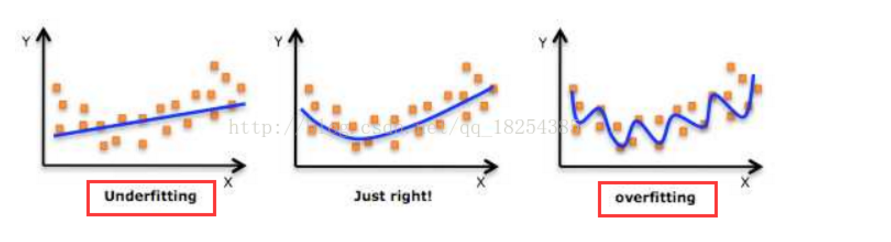过拟合的解决方法

1.数据清洗，得到较纯的数据。

一般在对数据进行训练前，首先会对数据进行处理，处理的具体方法后续章节补充。

2.增加训练的数据量。

3.采用正则化方法。

采用正则化方法是在损失函数后面加入正则化惩罚项因子，在这里λ我们称做正则化参数。而通过调整λ 这个正则化参数的大小，进而达成平衡拟合训练的目标和保持参数值较小的目标。当最小化J(θ)时，λ 越大,θ越小，所以通过调节λ的值可以调节θ的大小从而调节拟合的程度，λ过大会导致欠拟合，过小会导致过拟合。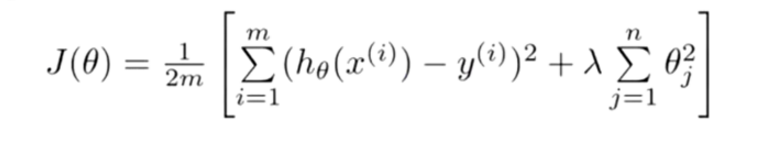4.采用dropout方法。

样本不均衡时解决方式

上采样/下采样

下采样，对于一个不均衡的数据，让目标值(如0和1分类)中的样本数据量相同，且以数据量少的一方的样本数量为准。上采样就是以数据量多的一方的样本数量为标准，把样本数量较少的类的样本数量生成和样本数量多的一方相同，称为上采样。

下采样

获取数据时一般是从分类样本多的数据从随机抽取等数量的yang样本。

人工合成数据策略

SMOTE全称是Synthetic Minority Oversampling Technique即合成少数类过采样技术。，SMOTE算法的基本思想是对少数类样本进行分析并根据少数类样本人工合成新样本添加到数据集中 。

算法流程如下： 1. 对于少数类中的每一个样本xx，用欧式距离为标准计算它到少数类样本集SminSmin中所有样本的距离，得到其k近邻 2. 确定采样倍率NN,对于每一个少数类样本xx,从其k近邻中随机选择若干个样本，假设选择的近邻为x^x^ 3. 对于每一个随机选出的近邻x^x^，分别与原样本按照如下的公式构建新的样本 xnew=x+rand(0,1)∗(x^−x)生成样本的数量和分类样本较多的数据量保持一致一致。
展开全文• 机器学习-样本不均衡或不足的处理办法 降采样采样 混合采样 代价敏感学习 样本不足
• 机器学习任务中，我们经常会遇到这种困扰：数据不平衡问题。 数据不平衡问题主要存在于有监督机器学习任务中。当遇到不平衡数据时，以总体分类准确率为学习目标的传统分类算法会过多地关注多数类，从而使得少数...
• 采样(under-sampling) ...在这样的数据分布的情况下，运用机器学习算法的预测模型可能会无法做出准确的预测，最后的模型显然是趋向于预测多数的，少数可能会被当做噪点或被忽视，相比多数，少数被...
• 其实我们在训练模型的过程，都会经常进行数据采样，为了就是让我们的模型可以更好的去学习数据的特征，从而让效果更佳。但这是比较浅层的理解，更本质上，数据采样就是对随机现象的模拟，根据给定的概率分布从而模拟...
• 这几年来，机器学习和数据挖掘非常火热，它们逐渐为世界带来实际价值。与此同时，越来越多的机器学习算法从学术界走向工业界，而在这个过程中会有很多困难。数据不平衡问题虽然不是最难的，但绝对是最...
• 本片博文主要是对机器学习的分类问题中存在的样本不均衡情况的处理说明，具体如下： 当对数据进行分类训练的时候，有时候会出现原始数据样本不均衡的情况，也就是正例与反例样本数量存在差异。此时为了能够更好的...
• from imblearn.over_sampling import ...# 对训练的数据进行上采样，测试的数据不需要SMOTE_train_x, SMOTE_train_y = overstamp.fit_sample(train_x, train_y) 由于数据分布的不均衡，因此对数据进行上采样，...
• 选自Analytics Vidhya 作者：Upasana Mukherjee ...如果你研究过一点机器学习和数据科学，你肯定遇到过不平衡的类分布（imbalanced class distribution）。这种情况是指：属于某一类别的观测样本的数量显著少
• 机器学习中的正负样本 所谓正样本（positive samples）、负样本（negative samples），对于某一环境下的人脸识别应用来说，比如教室中学生的人脸识别，则教室的墙壁，窗户，身体，衣服等等便属于负样本的范畴。 负...
• 采样： smote 假设有两个class：class1 和 class2 ，其中class1样本数少 从class1中随机选择一个点C，找到该点的K个邻居 从K个邻居中随机选择一个点C_ne 连接C与C1，在C与C_ne的连线上生成新的点C_new 重复1-...
• 机器学习任务中，我们经常会遇到这种困扰：数据不平衡问题。 数据不平衡问题主要存在于有监督机器学习任务中。当遇到不平衡数据时，以总体分类...绝大多数常见的机器学习算法对于不平衡数据都不能很好地工作。...
• 解决这一问题的基本思路是让正负样本在训练过程中拥有相同的话语权，比如利用采样与加权等方法。为了方便起见，我们把数据集中样本较多的那一类称为“大众类”，样本较少的那一类...采样方法是通过对训练进行处理...
• 1.背景 采样算法是机器学习中比较常用，也比较容易实现的（出去分层采样）。常用的采样算法有以下几种（来自百度知道）： 一、单纯随机抽样（simple random sampling） 将调查总体全部观察单位编号，再用抽签法或...
• 解决样本不均衡的问题很多，主流...1.过采样：将稀有类别的样本进行复制，通过增加此稀有类样本的数量来平衡数据。该方法适用于数据量较小的情况。 2.欠抽样：从丰富类别的样本中随机选取和稀有类别相同数目的样...
• 很多机器学习算法都有一个基本假设，就是数据分布是均匀的。当我们把这些算法直接应用在实际数据中时，大多数情况下都无法取得理想的结果，因为实际数据往往分布的很不均衡，都存在长尾效应。举个例子，大部分（假如...
...